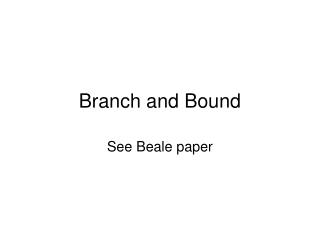DownloadDownload PresentationBranch and Bound

# Branch and Bound

Télécharger la présentation## Branch and Bound

- - - - - - - - - - - - - - - - - - - - - - - - - - - E N D - - - - - - - - - - - - - - - - - - - - - - - - - - -
##### Presentation Transcript

1. Branch and Bound See Beale paper

2. Solve First LP problem: • Solution is [1.5 2.5] x2 x1

3. [1.5 2.5] X1 >= 2 X1 <= 1 [2 1.5] , z=3.5 [1 1.5] , z=2.5 x2 x1

4. [1.5 2.5] X1 >= 2 X1 <= 1 [2 1.5] , z=3.5 [1 1.5] , z=2.5 X2<= 1 x2>= 2 [2.25, 1], z=3.25 No solution x2 x1

5. [2 1.5] , z=3.5 X2<= 1 x2>= 2 [1 1.5] , z=2.5 [2.25, 1], z=3.25 No solution X1 <= 2 x1 >= 3 [2,1], z=3 No solution x2 x1

6. S Sbar Sums edges out of S >= 2

7. In TSP, we solve LP problem with constraint {each vertex has 2 edges incident to it} and we add just relevant ‘subtour inequalities’ to cut off any subtour solutions. So each time we solve LP and if we get a subtour solution, we add the specific subtour inequality to cut off that solution and resolve LP. This continues until we get a final tour solution.

8. Unbounded Objective Function to be minimized

9. Infeasible solution

10. LP feasible, but integer infeasible